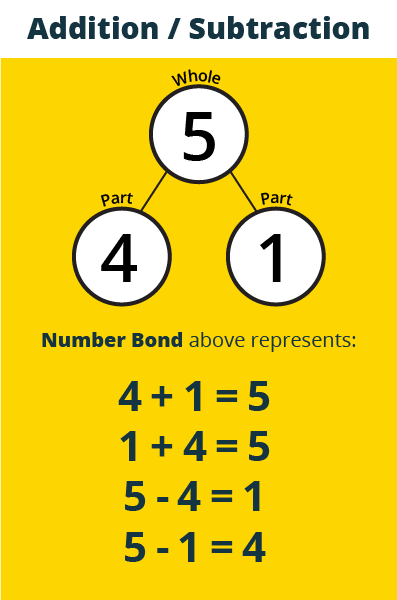# What is a Number Bond?

Many elementary schools teach math using Number Bonds, and it is super simple once you understand it.

A Number Bond is a visual representation of the relationship between addition and subtraction, showing the biggest number (the "whole") is made up of 2 smaller numbers (the "parts").# Benefits -- Algebraic Thinking

Instead of memorizing addition facts, practicing with number bonds help children get ready for algebra later on. When they look at a basic equation, they can recognize which of the numbers is the "whole" — the number at the top of the number bond — based on its position in the equation.

Example: x + 1 = 3

Kids who are used to number bonds would think of drawing a number bond with the "3" on top and put "1" in one of the circles at the bottom, and algebra will be easy and not scary when they get to it a few years later.